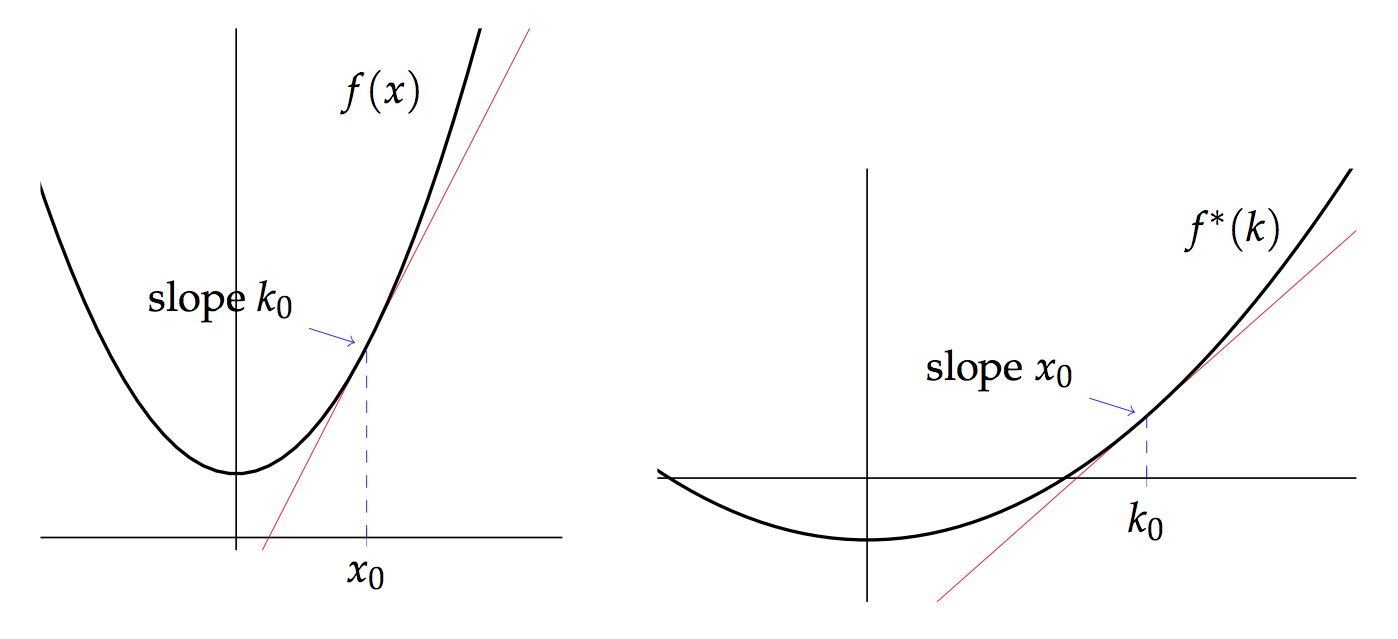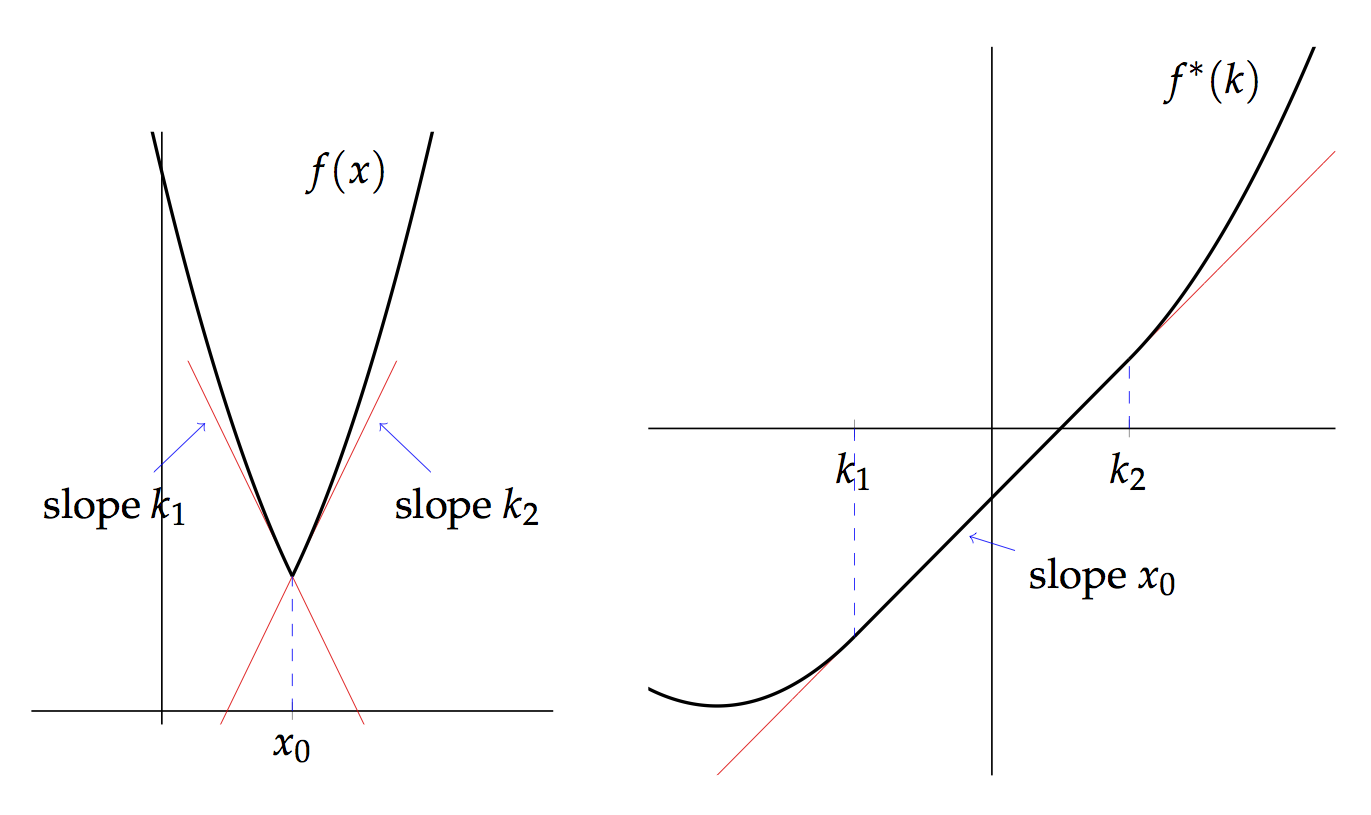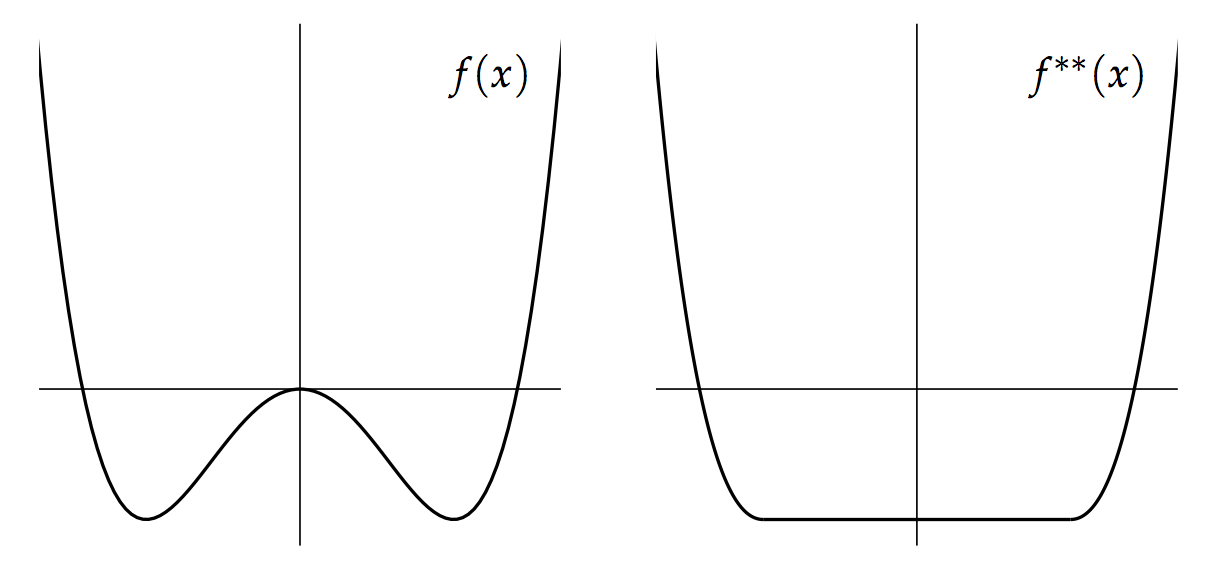## April 16, 2014

### Enrichment and the Legendre–Fenchel Transform I

#### Posted by Simon WillertonThe Legendre–Fenchel transform, or Fenchel transform, or convex conjugation, is, in its naivest form, a duality between convex functions on a vector space and convex functions on the dual space. It is of central importance in convex optimization theory and in physics it is used to switch between Hamiltonian and Lagrangian perspectives.Suppose that $V$ is a real vector space and that $f\colon V\to [-\infty ,+\infty ]$ is a function then the Fenchel transform is the function $f^{\ast }\colon V^{#}\to [-\infty ,+\infty ]$ defined on the dual vector space $V^{#}$ by $f^{\ast }(k)\coloneqq \sup _{x\in V}\big \{ \langle k,x\rangle -f(x)\big \} .$

If you’re a regular reader then you will be unsurprised when I say that I want to show how it naturally arises from enriched category theory constructions. I’ll show that in the next post. In this post I’ll give a little introduction to the Legendre–Fenchel transform.

There is probably no best way to introduce the Legendre–Fenchel transform. The only treatment that I knew for many years was in Arnold’s book Mathematical Methods of Classical Mechanics, but I have recently come across the convex optimization literature and would highly recommend Hugo Touchette’s The Fenchel Transform in a Nutshell — my treatment here is heavily influenced by this paper. I will talk mainly about the one-dimensional case as I think that gives a lot of the intuition.

We will start, as Legendre did, with the special case of a strictly convex differentiable function $f\colon \mathbb{R}\to \mathbb{R}$; for instance, the function $x^{2}+1/2$ pictured on the left hand side above. The derviative of $f$ is strictly increasing and so the function $f$ can be parametrized by the derivative $k =d f/d x$ instead of the parameter $x$. Indeed we can write the parameter $x$ in terms of the slope $k$, $x=x(k)$. The Legendre-Fenchel transform $f^{*}$ can then be defined to satisfy $\langle k,x \rangle = f(x) +f^{\ast }(k),$ where the angle brackets mean the pairing between a vector space and its dual. In this one-dimensional case, where $x$ and $k$ are thought of as real numbers, we just have $\langle k,x \rangle = k x$.

As $x$ is a function of $k$ we can rewrite this as $f^{\ast }(k)\coloneqq \langle k,x(k) \rangle -f(x(k)).$ So the Legendre-Fenchel transform encodes the function is a different way. By differentiating this equation you can see that the $d f^{\ast }/d k=x(k)$, thus we have interchanged the abcissa (the horizontal co-ordinate) and the slope. So if $f$ has derivative $k_{0}$ at $x_{0}$ then $f^{\ast }$ has derivative $x_{0}$ at $k_{0}$. This is illustrated in the above picture.

I believe this is what Legendre did and then that what Fenchel did was to generalize this to non-differentiable functions.

For non-differentiable functions, we can’t talk about tangent lines and derivatives, but instead can talk about supporting lines. A supporting line is one which touches the graph at at least one point and never goes above the graph. (The fact that we’re singling out lines not going above the graph means that we have convex functions in mind.)

For instance, at the point $(x_{0},f(x_{0}))$ the graph pictured below has no tangent line, but has supporting lines with gradient from $k_{1}$ to $k_{2}$. A convex function will have at least one supporting line at each point.It transpires that the right way to generalize the transform to this non-differentiable case is to define it as follows: $f^{\ast }(k)\coloneqq \sup _{x\in \mathbb{R}}\big \{ \langle k,x\rangle -f(x)\big \} .$ In this case, if $f$ has a supporting line of slope $k_{0}$ at $x_{0}$ then $f^{\ast }$ has a supporting line of slope $x_{0}$ at $k_{0}$. In the picture above, at $x_{0}$, the function $f$ has supporting lines with slope from $k_{1}$ to $k_{2}$: correspondingly, the function $f^{\ast }$ has supporting lines with slope $x_{0}$ all the way from $k_{1}$ to $k_{2}$.

If we allow the function $f$ to be not strictly convex then the transform will not alway be finite. For example, if $f(x)\coloneqq ax+b$ then we have $f^{\ast }(a)=-b$ and $f^{\ast }(k)=+\infty$ for $k\ne a$. So we will allow functions taking values in the extended real numbers: $\overline{\mathbb{R}}\coloneqq [-\infty ,+\infty ]$.

We can use the above definition to get the transform of any function $f\colon \mathbb{R}\to \overline{\mathbb{R}}$, whether convex or not, but the resulting transform $f^{\ast }$ is always convex. (When there are infinite values involved we can also say that $f^{\ast }$ is lower semi-continuous, but I’ll absorb that into my definition of convex for functions taking infinite values.)

Everything we’ve done for one-dimensional $\mathbb{R}$ easily generalizes to any finite dimensional real vector space $V$, where we should say ‘supporting hyperplane’ instead of ‘supporting line’. From that we get a transform between sets of functions $(\text {--})^{\ast }\colon \mathrm{Fun}(V,\overline{\mathbb{R}})\to \mathrm{Fun}(V^{#},\overline{\mathbb{R}}),$ where $V^{#}$ is the vector space dual of $V$. Similarly, we have a reverse transform going the other way, which is traditionally also denoted with a star $(\text {--})^{\ast }\colon \mathrm{Fun}(V^{#},\overline{\mathbb{R}})\to \mathrm{Fun}(V,\overline{\mathbb{R}}),$ for $g\colon V^{#}\to \overline{\mathbb{R}}$ we define $g^{\ast }(x)\coloneqq \sup _{k\in V^{#}}\big \{ \langle k,x\rangle -g(k)\big \} .$

This pair of transforms have some rather nice properties, for instance, they are order reversing. We can put a partial order on any set of functions to $\overline{\mathbb{R}}$ by defining $f_{1}\ge f_{2}$ if $f_{1}(x)\ge f_{2}(x)$ for all $x$. Then $f_{1}\ge f_{2} \quad \Rightarrow \quad f_{2}^{\ast }\ge f_{1}^{\ast }.$ Also for any function $f$ we have $f^{\ast }=f^{\ast \ast \ast }$ which implies that the operator $f\mapsto f^{\ast \ast }$ is idempotent: $f^{\ast \ast }=f^{\ast \ast \ast \ast }.$ This means that $f\mapsto f^{\ast \ast }$ is a closure operation. What it actually does is take the convex envelope of $f$, which is the largest convex function less than or equal to $f$. Here’s an example.This gives that if $f$ is already a convex function then $f^{\ast \ast }=f$. And as a consequence the Legendre–Fenchel transform and the reverse transform restrict to an order reversing bijection between convex functions on $V$ and convex functions on its dual $V^{#}$. $\mathrm{Cvx}(V,\overline{\mathbb{R}})\cong \mathrm{Cvx}(V^{#},\overline{\mathbb{R}}).$

There are many other things that can be said about the transform, such as Fenchel duality and the role it plays in optimization, but I don’t understand such things to my own satisfaction yet.

Next time I’ll explain how most of the above structure drops out of the nucleus construction in enriched category theory.

Posted at April 16, 2014 10:59 AM UTC

TrackBack URL for this Entry:   https://golem.ph.utexas.edu/cgi-bin/MT-3.0/dxy-tb.fcgi/2732

### Re: Enrichment and the Legendre-Fenchel Transform I

Physics question: what’s the quantum counterpart to one’s classical Hamiltonian being convex?

I.e. what property does the “corresponding” Hermitian operator on $L^2(R^n)$ have?

Posted by: Allen Knutson on April 16, 2014 3:30 PM | Permalink | Reply to this

### Re: Enrichment and the Legendre-Fenchel Transform I

This is interesting. I wonder how it relates to the “span” or “Grassmannian” viewpoint of the Legendre transform. I’ve forgotten how exactly that works, but it expresses the Legendre transform as a kind of duality between planes and hyperplanes.

Here is a nice physics paper on the “bog-standard” Legendre transform in case anyone is interested: Making sense of the Legendre transform. Though it doesn’t answer Allen’s question.

Posted by: Bruce Bartlett on April 16, 2014 6:29 PM | Permalink | Reply to this

### Re: Enrichment and the Legendre-Fenchel Transform I

Allen, a slight cop out of an answer would be that the corresponding quantum Hamiltonian will be a pseudo-differential operator with a convex principal symbol. Off the top of my head I’m not sure how the convexity of the principal symbol translates into more abstract operator-theoretic properties.

Bruce, you might be thinking of the so called polar transform. Given a vector space $V$ and any subset $S$, you can consider all oriented codimension-1 hyperplanes whose positive half space contains $S$. These hyperplanes are in 1-1 correspondence with non-zero elements of the dual vector space $V^*$, via the equation $\langle k, x\rangle \ge 1$. So, the duality is between hyperplanes and points of the dual space. The polar transform of $S$ is $S^* = \{ k \in V^* \mid \langle k, x\rangle \ge 1, x\in S \}$. The polar transform has nice properties like preserving convexity, reversing inclusions, mapping between $l^p$ and $l^q$ unit balls, …

If we replace $V$ by $V\times \mathbb{R}$ and take $S$ as the graph of a function $f\colon V\to \mathbb{R}$, then the boundary of the polar dual $S^* \subseteq V^* \times \mathbb{R}$ is the graph of the Legendre transform $f^*\colon V^* \to \mathbb{R}$.

Another interesting property of the Legendre transform is its use in semiclassical asymptotics. If $f(x)$ is convex and $f^*(k)$ is its Legendre transform, then the Fourier transform of $F(x) = \exp( i f(x) )$ is equal to $\tilde{F}(k) = \exp( -i f^*(k) + err)$, with “err” being exactly zero for quadratic polynomials.

Posted by: Igor Khavkine on April 17, 2014 3:23 PM | Permalink | Reply to this

### Re: Enrichment and the Legendre-Fenchel Transform I

Thanks Igor, yes the polar transform indeed sounds like the “hyperplane” take on the Legendre transform I was thinking of.

Posted by: Bruce Bartlett on April 21, 2014 10:58 PM | Permalink | Reply to this

### Re: Enrichment and the Legendre-Fenchel Transform I

Great! Are we going to get to find out the commonality between the Legendre and Laplace transforms (and others)?

Posted by: David Corfield on April 18, 2014 3:41 PM | Permalink | Reply to this

### Re: Enrichment and the Legendre-Fenchel Transform I

David, the asymptotic formula for Fourier transforms is an instance of of the saddle point or steepest descent method. That method applies also to integrals of exponentials with real arguments as well. Namely for convex $f(x)$, the Laplace transform of $F(x) = \exp(-f(x))$ is $\tilde{F}(x) = \exp(f^*(x) + err)$. I’ve not inserted any overall constant or limits. These can be obtained as an exercise in applying the steepest descent approximation.

Posted by: Igor Khavkine on April 18, 2014 4:38 PM | Permalink | Reply to this

### Re: Enrichment and the Legendre-Fenchel Transform I

I’m remembering a question I heard Alan Weinstein ask about this at some lecture. He referred to “the classical Legendre transform”. You might think that’s the one defined here. But no! this one is the “(quantum) Legendre transform”, since it’s about functions on vector spaces, hence quantum objects.

Posted by: Allen Knutson on April 20, 2014 1:30 AM | Permalink | Reply to this

### Re: Enrichment and the Legendre-Fenchel Transform I

That sounds interesting. Do you remember what was meant by “the classical Legendre transform”?

Posted by: Simon Willerton on April 20, 2014 1:58 PM | Permalink | Reply to this

### Re: Enrichment and the Legendre–Fenchel Transform I

Here is a bit of noodling around that I found useful when I first thought about Legendre transforms.

Let $V$ be a finite dimension real vector space and $f: V \to \mathbb{R}$ be a convex function. Then $df$ is a map from $V$ to $V^{\vee}$, the dual vector space. Conversely, $df^{\ast}$ is a map from $V^{\vee}$ to $V$. These two maps are inverse. Simon describes this for one dimensional maps – “if $f$ has derivative $k_{0}$ at $x_{0}$ then $f^{\ast}$ has derivative $x_{0}$ at $k_{0}$” – but it is right in any dimension.

If we didn’t know about the Legendre transform, this would seem like a pretty amazing fact about differential forms: If $\omega$ is an exact form on $V$, then $\omega^{-1}$ is an exact form on $V^{\vee}$, where the inverse means that we think of $\omega$ as a map $V \to V^{\vee}$ and then invert it. Since $V$ and $V^{\vee}$ are simply connected, we can ask the question instead about closed forms. Writing $\omega = \sum g_i dx_i$, the condition that $\omega$ is closed is that $\frac{\partial g_i}{\partial x_j} = \frac{\partial g_j}{\partial x_i}$. I.e. the matrix $\left(\frac{\partial g_i}{\partial x_j} \right)$ should be symmetric. The amazing fact turns into the quite simple fact that the inverse of a symmetric matrix is also symmetric.

Hate matrices? We can also state this in a basis free way. Put a skew-symmetric form on $V \oplus V^{\ast}$ in the standard way. Then the condition that $\omega$ is closed says that the graph of $\omega: V \to V^{\ast}$ is a Lagrangian subspace; if we instead consider the graph of $\omega^{-1} : V^{\ast} \to V$, we are considering the same space.

Posted by: David Speyer on April 21, 2014 2:07 PM | Permalink | Reply to this

### Re: Enrichment and the Legendre–Fenchel Transform I

The nutshell paper has gone missing from Tourchette’s webpage, but is still available on the wayback machine.

Posted by: Mike Stay on October 14, 2014 1:29 AM | Permalink | Reply to this

### Re: Enrichment and the Legendre–Fenchel Transform I

Thanks Mike. I’ve updated the link to his current page in Stellenbosch. I’ve also corrected my misspelling of his name to Touchette.

Posted by: Simon Willerton on October 14, 2014 5:06 PM | Permalink | Reply to this

### Re: Enrichment and the Legendre–Fenchel Transform I

It seems that the link to the “Legendre transform in a nutshell” text has been changed again, here’s what seems to be the current one: http://www.physics.sun.ac.za/~htouchette/archive/notes/lfth2.pdf

Posted by: Alex Makelov on March 15, 2017 1:15 AM | Permalink | Reply to this

### Re: Enrichment and the Legendre–Fenchel Transform I

Posted by: Simon Willerton on March 15, 2017 8:36 AM | Permalink | Reply to this

### Re: Enrichment and the Legendre–Fenchel Transform I

Now:

http://appliedmaths.sun.ac.za/~htouchette/archive/notes/lfth2.pdf

Posted by: Arthur on December 24, 2019 9:51 AM | Permalink | Reply to this

### Re: Enrichment and the Legendre–Fenchel Transform I

Posted by: Simon Willerton on May 11, 2021 9:28 AM | Permalink | Reply to this

Post a New Comment